ID 935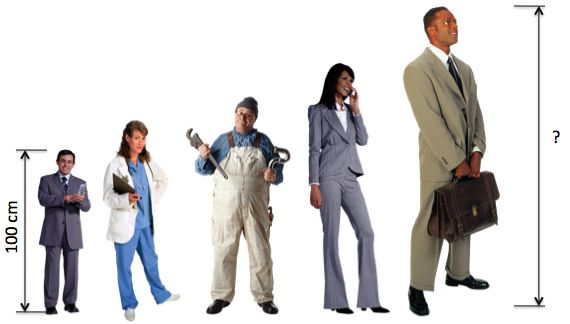The first person is 100 cm tall.
Each subsequent person is 20% taller than the person before.

What will be the height of the fifth person?

ID 966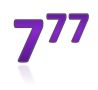The last digit of the number 777 is

ID 993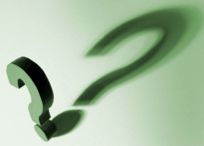Find the hundreds digit (third digit from the right) of the product:

44444 x 99999

ID 1000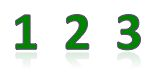Which gives the largest answer?

ID 1274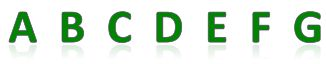Which is the letter D in the row?

ID 1282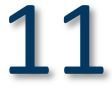What number is 3 greater than 11?

ID 1880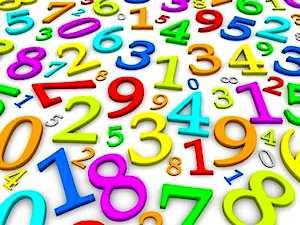Which is the sum of all digits of the decimal representation of 516 x 165?

ID 2032The sum of the digits of a two-digit number X is Y.
The sum of the digits of X2 is Y2.
Find X.

ID 2145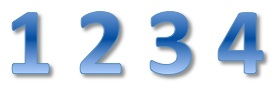Which gives the largest answer?

ID 2225How many three-digit numbers have exactly five divisors including 1 and itself?

ID 2242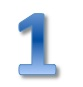Make two fractions with all the digits from 0 to 9.
Use each digit once.
They add up to exactly 1.

What is one of the fractions?

ID 3541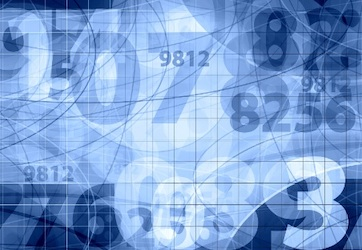What is 2 to the power of 16?

ID 3719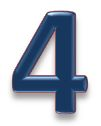If you wrote all the whole numbers from 1 through 1000, how many times would you write the digit 4?

ID 3743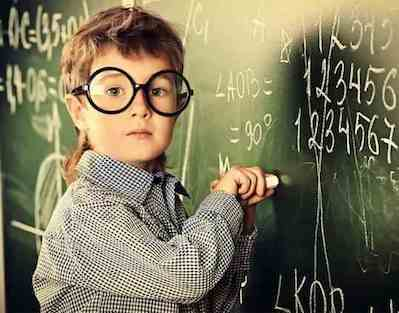With a 1 after a nine-digit number, it is the same as a 1 before it.

Find the sum of its digits.

ID 3794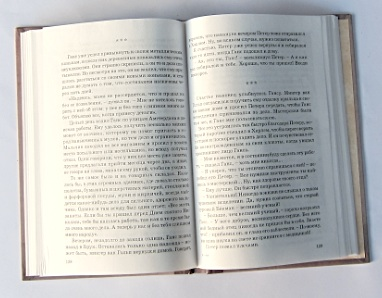I multiply the two page numbers and get 6162.

What is the right-hand page number?

ID 3846What are the two last digits of the result?
1999999991 x 2000000009

ID 4025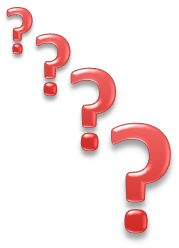If XY = 1024 and both numbers are integers, which of the following could not be a value of X nor Y?

ID 4091Which number is smaller than the sum of its divisors other than 1 and the number itself?

ID 4160Which numeral system states that

7 + 6 = 15

ID 4167How many digits are there in the number 62510?

Please don't use a calculator or counting apps.

ID 4378Which of the following gives the largest result?

Do not use a calculator.

ID 4687Savin's problem:

Using each of the digits 1,2,3, and 4 twice, write out an eight-digit number in which there is one digit between the ones, two digits between the twos, three digits between the threes, and four digits between the fours.
How many such numbers?

ID 4705What gives the smallest result?

Here we write 0.333 . . . as notation for 0.3 recurring.

ID 4786What is the sum of the first 100 prime numbers?

A prime number is a natural number greater than 1 that has no positive divisors other than 1 and itself.

ID 5001The average of 99 consecutive numbers is 99.
What is the average of the nine smallest of these numbers?

ID 5060The natural numbers are the counting numbers, like 1, 2, 3, et cetera.

An even number is a natural number which is "evenly divisible" by two.

An odd number is a natural number which is not a multiple of two.

Which statement is correct?

ID 5061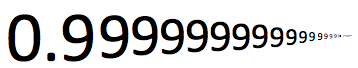0.999 . . . means infinitely repeating decimals.

What is the largest result?

ID 5140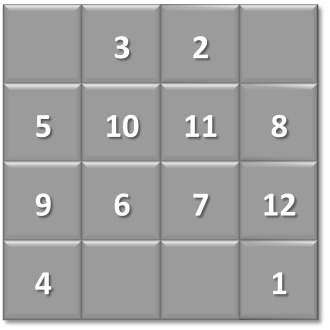A magic square is an arrangement of integers, in a square grid, where the numbers in each row, and in each column, and the numbers in the diagonals, all add up to the same number.

Place numbers 13, 14, 15, and 16 in the square to make a magic square.

Which number do you place in the top left cell?

ID 5222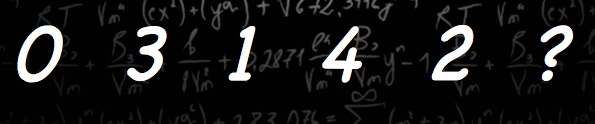Leslie Green asks:

What is the next number in the sequence?

0, 3, 1, 4, 2

(NOTE: It is a single sequence and not two sequences interleaved.)

ID 5426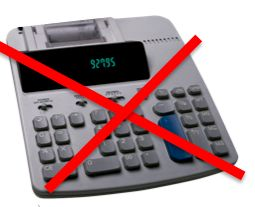Shakuntala Devi was undoubtedly the most brilliant arithmetic mental calculator of all time. In 1977 she mentally calculated the 23rd root of a 201 digit number in a mere 50 seconds. She toured the world showing how she could do calculations faster than they could be entered into and solved by the computers of the day.

The problem for you is much simpler: Evaluate (without using a calculator) the 20th root of the 11 digit number consisting of 1 followed by all zeros.

Author: Leslie Green

ID 5836ONE + ONE =

You can add zero(s) where you want.

What can be the result?

ID 5897The product of any 4 consecutive positive integers is always divisible by

ID 6219The numbers

129
CXXIX
10000001
81

all have the same value.

Why is CXXIX the odd one out?

Author: Leslie Green

ID 6286What digit is the most frequent between the numbers 1 and 1,000,000 inclusive?

ID 6762Present 100 as a sum of positive integers such that their product is at its maximum value.

How many numbers do you use?